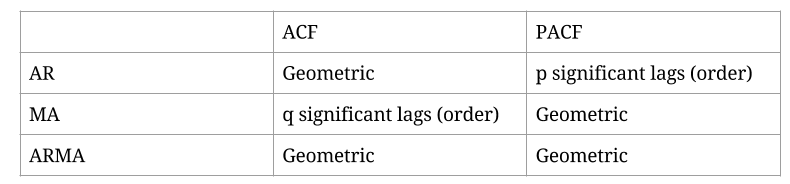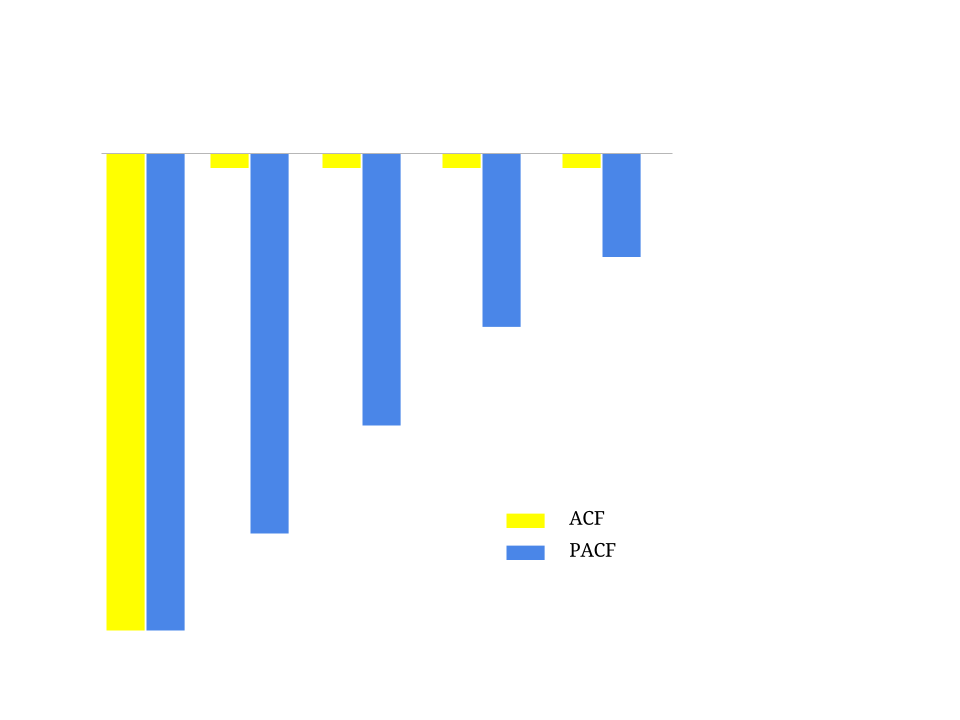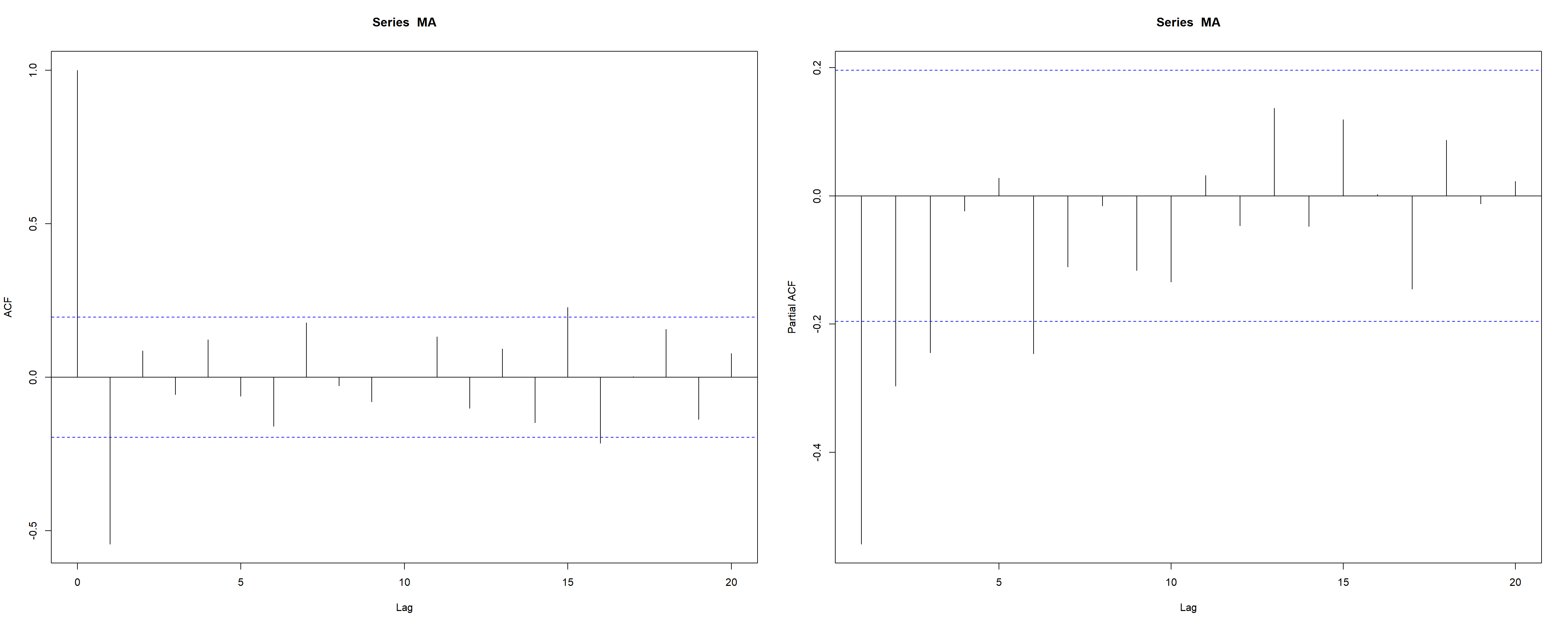### Time Series Analysis From Plots:

From this youtube post. Also, here is a more extensive document with simulations found online.Examples:

#### Moving Average MA(1):On this plot the ACF is significant only once (in reality the first entry in the ACF is always significant, since there is no lag in the first entry - it’s the correlation with itself), while the PACF is geometric. Hence it is an MA(1) process.

The negative values in the plot respond to a process of the form $$\large\color{blue} {y_t} = k - \color{red}{\theta}\,\epsilon_{t-1}+\epsilon_t.$$

Here is a simulation of an MA(1) process with $$\theta = - 0.7:$$

set.seed(2017)
MA = arima.sim(model=list(ma = - 0.7), n = 100)
par(mfrow = c(1,2)); acf(MA); pacf(MA)par(mfrow=c(1,1))
library(ggplot2)
library(fpp2)
ggtsdisplay(MA)